### Home > INT3 > Chapter Ch11 > Lesson 11.1.1 > Problem11-7

11-7.
1. Simplify the expressions below. Homework Help ✎

2.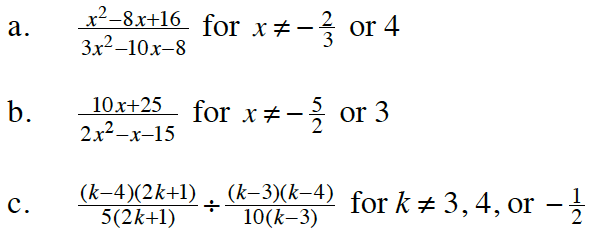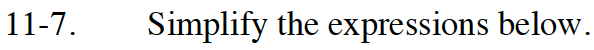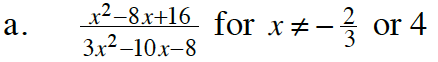Factor the numerator and denominator.

$\frac{\left(x-4\right)\left(x-4\right)}{\left(3x+2\right)\left(x-4\right)}$

After factoring, look for a Giant One to remove.

$\frac{x-4}{3x+2}$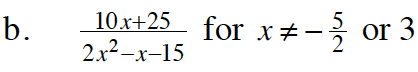Refer to part (a).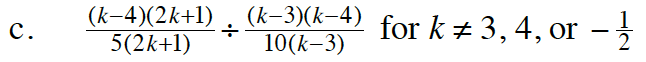This is division with fractions. Here is a sample division problem with numbers.

$\frac{6}{5}\div\frac{24}{5}$

Step 1: Rewrite as multiplication by taking the reciprocal of the divisor.

$\frac{6}{5}\cdot\frac{5}{24}$

Step 2: Factor 24 and use the commutative property to rewrite the problem so
there are Giant Ones.

$\frac{5}{5}\cdot\frac{6\cdot1}{6\cdot4}$

Step 3: Remove the Giant Ones.

Apply these steps to part (c).

$\frac{1}{4}$